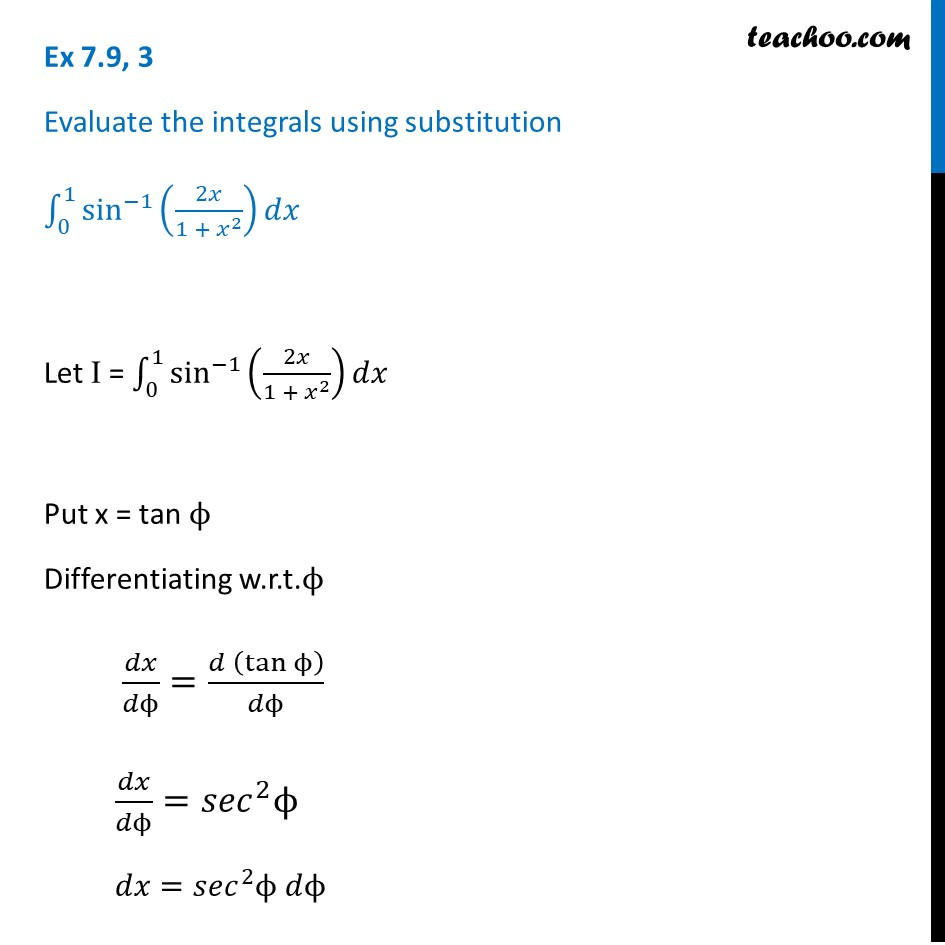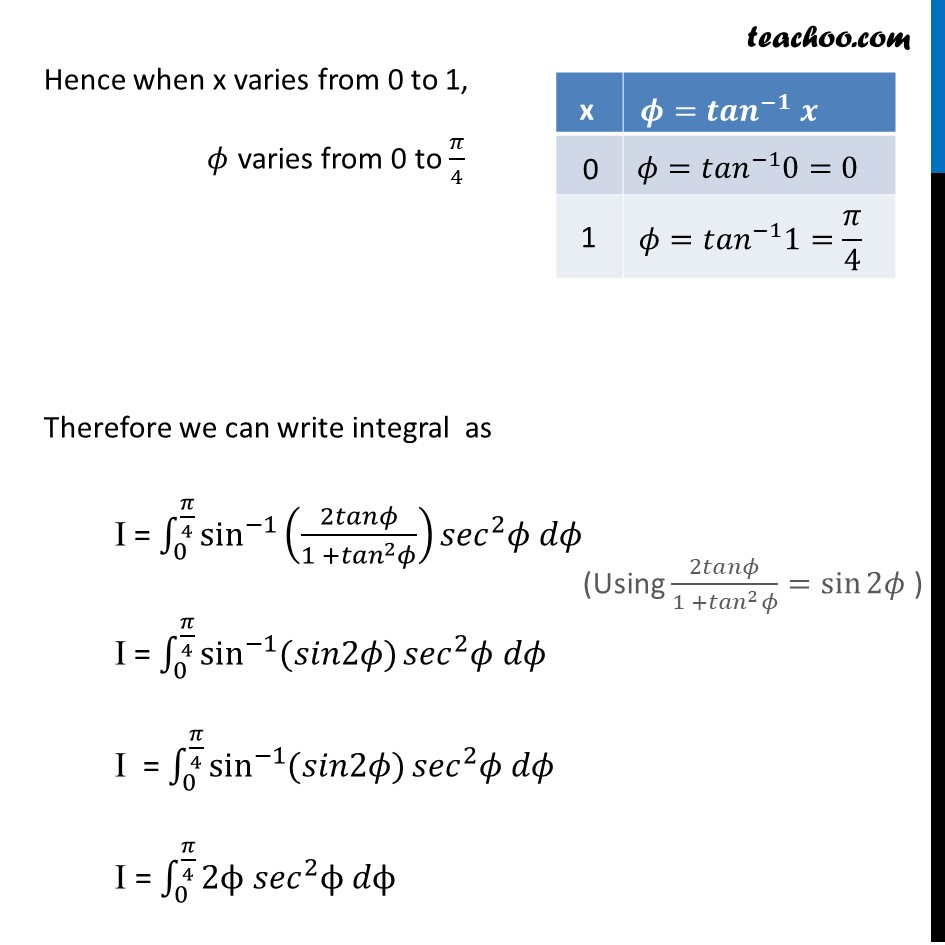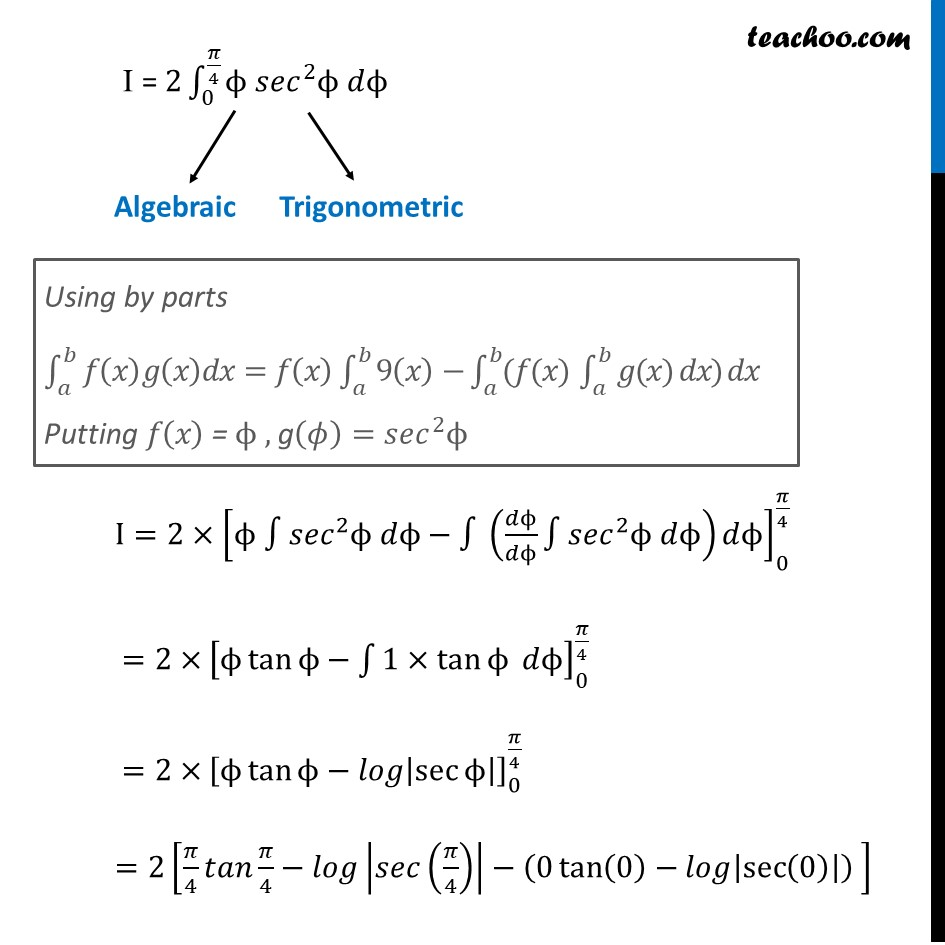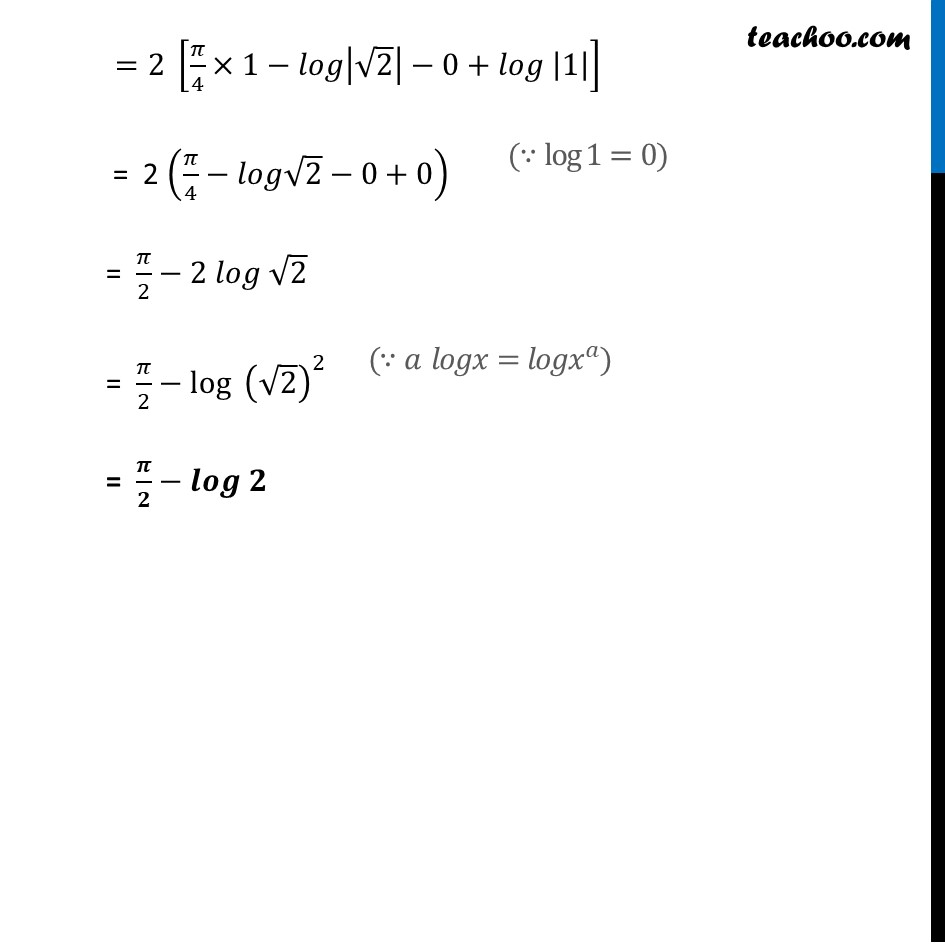Ex 7.9

Chapter 7 Class 12 Integrals
Serial order wiseLearn in your speed, with individual attention - Teachoo Maths 1-on-1 Class

### Transcript

Ex 7.9, 3 Evaluate the integrals using substitution ∫_0^1▒sin^(−1)⁡(2𝑥/(1 + 𝑥^2 )) 𝑑𝑥 Let I = ∫_0^1▒sin^(−1)⁡(2𝑥/(1 + 𝑥^2 )) 𝑑𝑥 Put x = tan ϕ Differentiating w.r.t.ϕ 𝑑𝑥/𝑑ϕ=(𝑑 (tan⁡ϕ ))/𝑑ϕ 𝑑𝑥/𝑑ϕ=〖𝑠𝑒𝑐〗^2 ϕ 𝑑𝑥=〖𝑠𝑒𝑐〗^2 ϕ 𝑑ϕ Hence when x varies from 0 to 1, 𝜙 varies from 0 to 𝜋/4 Therefore we can write integral as I = ∫_0^(𝜋/4)▒sin^(−1)⁡(2𝑡𝑎𝑛𝜙/(1 +〖𝑡𝑎𝑛〗^2 𝜙)) 〖𝑠𝑒𝑐〗^2 𝜙 𝑑𝜙 I = ∫_0^(𝜋/4)▒sin^(−1)⁡〖(𝑠𝑖𝑛2𝜙)〗 〖𝑠𝑒𝑐〗^2 𝜙 𝑑𝜙 I = ∫_0^(𝜋/4)▒sin^(−1)⁡〖(𝑠𝑖𝑛2𝜙)〗 〖𝑠𝑒𝑐〗^2 𝜙 𝑑𝜙 I = ∫_0^(𝜋/4)▒〖2ϕ 〖𝑠𝑒𝑐〗^2 ϕ 𝑑ϕ" " 〗 I = 2∫_0^(𝜋/4)▒〖ϕ 〖𝑠𝑒𝑐〗^2 ϕ 𝑑ϕ" " 〗 I=2×[ϕ ∫〖𝑠𝑒𝑐〗^2 ϕ 𝑑ϕ−∫(𝑑ϕ/𝑑ϕ∫〖𝑠𝑒𝑐〗^2 ϕ 𝑑ϕ)𝑑ϕ]_0^(𝜋/4) =2× [ϕ tan⁡ϕ−∫1▒1×tan⁡ϕ 𝑑ϕ]_0^(𝜋/4) =2× [ϕ tan⁡ϕ−𝑙𝑜𝑔|sec⁡ϕ |]_0^(𝜋/4) =2[𝜋/4 𝑡𝑎𝑛 𝜋/4−𝑙𝑜𝑔|𝑠𝑒𝑐(𝜋/4)|−(0 tan⁡(0)−𝑙𝑜𝑔|sec⁡(0) |) ] =2 [𝜋/4×1−𝑙𝑜𝑔|√2|−0+𝑙𝑜𝑔 |1|] = 2 (𝜋/4−𝑙𝑜𝑔√2−0+0) = 𝜋/2−2 𝑙𝑜𝑔 √2 = 𝜋/2−log⁡〖 (√2)^2 〗 = 𝝅/𝟐−𝒍𝒐𝒈 𝟐